Publicité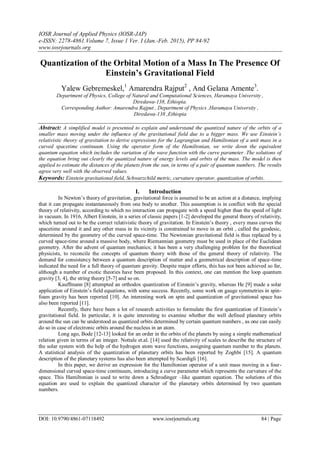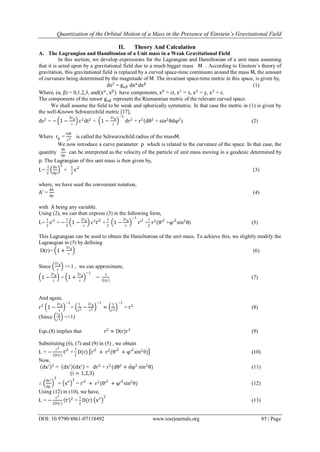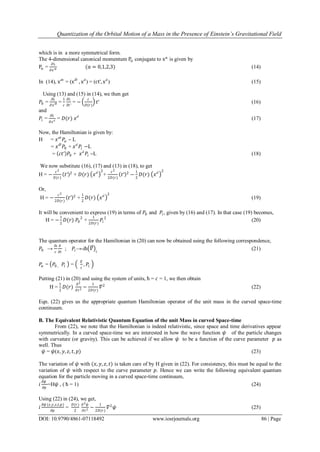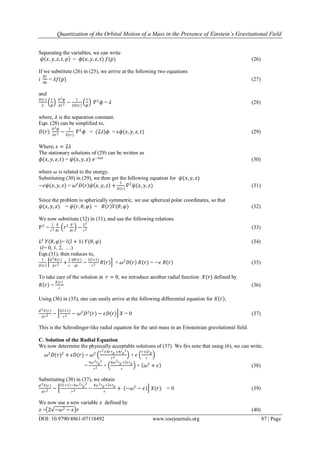Publicité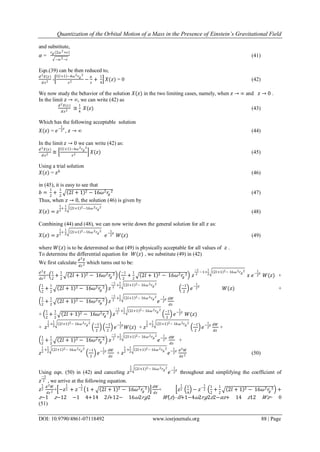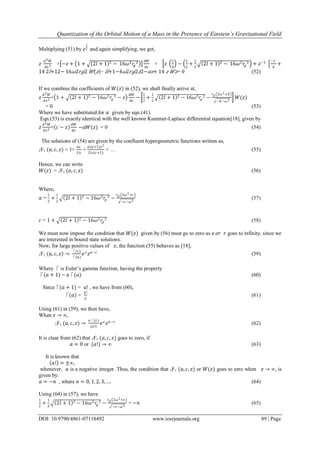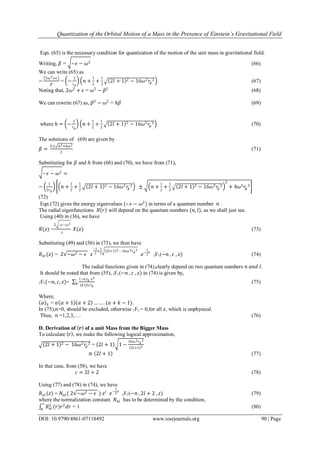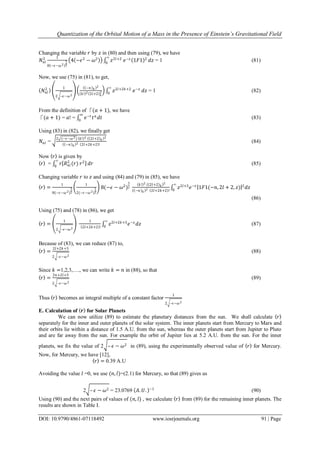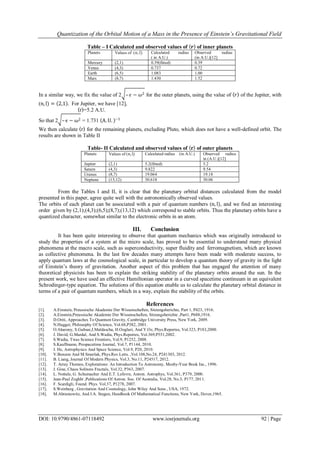Prochain SlideShare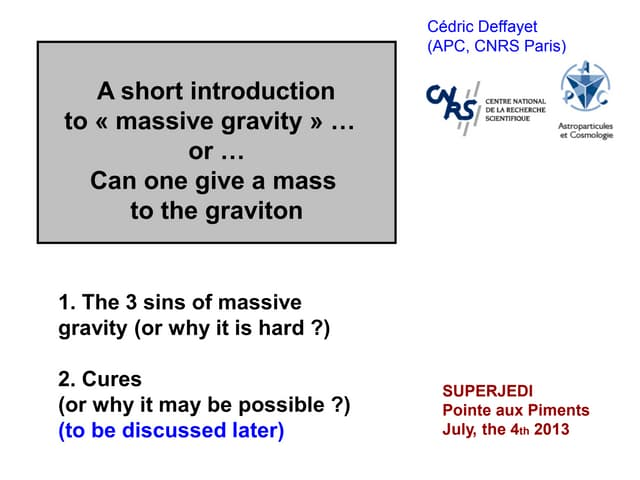A short introduction to massive gravity... or ... Can one give a mass to the ...
Chargement dans ... 3
1 sur 9
Publicité

### Quantization of the Orbital Motion of a Mass In The Presence Of Einstein’s Gravitational Field

1. IOSR Journal of Applied Physics (IOSR-JAP) e-ISSN: 2278-4861.Volume 7, Issue 1 Ver. I (Jan.-Feb. 2015), PP 84-92 www.iosrjournals.org DOI: 10.9790/4861-07118492 www.iosrjournals.org 84 | Page Quantization of the Orbital Motion of a Mass In The Presence Of Einstein’s Gravitational Field Yalew Gebremeskel,1 Amarendra Rajput2 , And Gelana Amente3 . Department of Physics, College of Natural and Computational Sciences, Haramaya University , Diredawa-138, Ethiopia. Corresponding Author: Amarendra Rajput , Department of Physics ,Haramaya University , Diredawa-138 ,Ethiopia Abstract: A simplified model is presented to explain and understand the quantized nature of the orbits of a smaller mass moving under the influence of the gravitational field due to a bigger mass. We use Einstein’s relativistic theory of gravitation to derive expressions for the Lagrangian and Hamiltonian of a unit mass in a curved spacetime continuum. Using the operator form of the Hamiltonian, we write down the equivalent quantum equation which includes the variation of the wave function with the curve parameter .The solutions of the equation bring out clearly the quantized nature of energy levels and orbits of the mass. The model is then applied to estimate the distances of the planets from the sun, in terms of a pair of quantum numbers. The results agree very well with the observed values. Keywords: Einstein gravitational field, Schwarzchild metric, curvature operator, quantization of orbits. I. Introduction In Newton’s theory of gravitation, gravitational force is assumed to be an action at a distance, implying that it can propagate instantaneously from one body to another. This assumption is in conflict with the special theory of relativity, according to which no interaction can propagate with a speed higher than the speed of light in vacuum. In 1916, Albert Einstein, in a series of classic papers [1-2] developed the general theory of relativity, which turned out to be the correct relativistic theory of gravitation. In Einstein’s theory , every mass curves the spacetime around it and any other mass in its vicinity is constrained to move in an orbit , called the geodesic, determined by the geometry of the curved space-time. The Newtonian gravitational field is thus replaced by a curved space-time around a massive body, where Riemannian geometry must be used in place of the Euclidean geometry. After the advent of quantum mechanics; it has been a very challenging problem for the theoretical physicists, to reconcile the concepts of quantum theory with those of the general theory of relativity. The demand for consistency between a quantum description of matter and a geometrical description of space-time indicated the need for a full theory of quantum gravity. Despite major efforts, this has not been achieved so far, although a number of exotic theories have been proposed. In this context, one can mention the loop quantum gravity [3, 4], the string theory [5-7] and so on. Kauffmann  attempted an orthodox quantization of Einstein’s gravity, whereas He  made a solar application of Einstein’s field equations, with some success. Recently, some work on gauge symmetries in spin- foam gravity has been reported . An interesting work on spin and quantization of gravitational space has also been reported . Recently, there have been a lot of research activities to formulate the first quantization of Einstein’s gravitational field. In particular, it is quite interesting to examine whether the well defined planetary orbits around the sun can be understood as quantized orbits determined by certain quantum numbers , as one can easily do so in case of electronic orbits around the nucleus in an atom. Long ago, Bode [12-13] looked for an order in the orbits of the planets by using a simple mathematical relation given in terms of an integer. Nottale et.al.  used the relativity of scales to describe the structure of the solar system with the help of the hydrogen atom wave functions, assigning quantum number to the planets. A statistical analysis of the quantization of planetary orbits has been reported by Zoghbi . A quantum description of the planetary systems has also been attempted by Scardigli . In this paper, we derive an expression for the Hamiltonian operator of a unit mass moving in a four- dimensional curved space-time continuum, introducing a curve parameter which represents the curvature of the space. This Hamiltonian is used to write down a Schrodinger –like quantum equation. The solutions of this equation are used to explain the quantized character of the planetary orbits determined by two quantum numbers.
2. Quantization of the Orbital Motion of a Mass in the Presence of Einstein’s Gravitational Field DOI: 10.9790/4861-07118492 www.iosrjournals.org 85 | Page II. Theory And Calculation A. The Lagrangian and Hamiltonian of a Unit mass in a Weak Gravitational Field In this section, we develop expressions for the Lagrangian and Hamiltonian of a unit mass assuming that it is acted upon by a gravitational field due to a much bigger mass M . According to Einstein’s theory of gravitation, this gravitational field is replaced by a curved space-time continuum around the mass M, the amount of curvature being determined by the magnitude of M. The invariant space-time metric in this space, is given by, ds2 = gαβ dxα dxβ (1) Where, (α, β) = 0,1,2,3, and(xα , xβ ) have components, x0 = ct, x1 = x, x2 = y, x3 = z. The components of the tensor gαβ represent the Riemannian metric of the relevant curved space. We shall assume the field to be weak and spherically symmetric. In that case the metric in (1) is given by the well-Known Schwarzchild metric , ds2 = − 1 − 2rg r c2 dt2 + 1 − 2rg r −1 dr2 + r2 (dθ2 + sin2 θdφ2 ) (2) Where rg = GM c2 is called the Schwarzschild radius of the massM. We now introduce a curve parameter p which is related to the curvature of the space. In that case, the quantity ds dp can be interpreted as the velocity of the particle of unit mass moving in a geodesic determined by p. The Lagrangian of this unit mass is then given by, L= 1 2 ds dp 2 = 1 2 s′2 (3) where, we have used the convenient notation, A′ = dA dp (4) with A being any variable. Using (2), we can then express (3) in the following form, L= 1 2 s′2 = − 1 2 1 − 2rg r c2 t′2 + 1 2 1 − 2rg r −1 r′2 + 1 2 r2 (θ′2 +φ′2 sin2 θ) (5) This Lagrangian can be used to obtain the Hamiltonian of the unit mass. To achieve this, we slightly modify the Lagrangian in (5) by defining D(r)= 1 + 2rg r (6) Since 2rg r <<1 , we can approximate, 1 − 2rg r = 1 + 2rg r −1 = 1 D(r) (7) And again, r2 1 − 2rg r −1 = 1 r2 − 2rg r3 −1 ≈ 1 r2 −1 = r2 (8) (Since rg r3 <<1) Eqn.(8) implies that r2 ≈ D(r)r2 (9) Substituting (6), (7) and (9) in (5) , we obtain L = − c2 2D(r) t′2 + 1 2 D(r) r′2 + r2 (θ′2 + φ′2 sin2 θ) (10) Now, dxi 2 = dxi dxi = dr2 + r2 (dθ2 + dφ2 sin2 θ) (11) (i = 1,2,3) ∴ dxi dp 2 = x′i 2 = r′2 + r2 (θ′2 + φ′2 sin2 θ) (12) Using (12) in (10), we have, L = − c2 2D(r) t′ 2 + 1 2 D(r) x′i 2 (13)
3. Quantization of the Orbital Motion of a Mass in the Presence of Einstein’s Gravitational Field DOI: 10.9790/4861-07118492 www.iosrjournals.org 86 | Page which is in a more symmetrical form. The 4-dimensional canonical momentum Pα conjugate to xα is given by Pα = ∂L ∂x′α (α = 0,1,2,3) (14) In (14), x′α = (x′0 , x′i ) = (ct′, x′i ) (15) Using (13) and (15) in (14), we then get 𝑃0 = 𝜕𝐿 𝜕𝑥′0 = 1 𝑐 𝜕𝐿 𝜕𝑡′ = − 𝑐 𝐷(𝑟) 𝑡′ (16) and 𝑃𝑖 = 𝜕𝐿 𝜕𝑥′𝑖 = 𝐷(𝑟) 𝑥′𝑖 (17) Now, the Hamiltonian is given by: H = 𝑥′ 𝛼 𝑃𝛼 – L = 𝑥′0 𝑃0 + 𝑥′𝑖 𝑃𝑖 −L = 𝑐𝑡′ 𝑃0 + 𝑥′𝑖 𝑃𝑖 –L (18) We now substitute (16), (17) and (13) in (18), to get H = − 𝑐2 𝐷(𝑟) 𝑡′ 2 + 𝐷(𝑟) 𝑥′𝑖 2 + 𝑐2 2𝐷(𝑟) 𝑡′ 2 − 1 2 𝐷(𝑟) 𝑥′𝑖 2 Or, H = − 𝑐2 2𝐷(𝑟) 𝑡′ 2 + 1 2 𝐷(𝑟) 𝑥′𝑖 2 (19) It will be convenient to express (19) in terms of 𝑃0 and 𝑃𝑖, given by (16) and (17). In that case (19) becomes, H = − 1 2 𝐷(𝑟) 𝑃0 2 + 1 2𝐷(𝑟) 𝑃𝑖 2 (20) The quantum operator for the Hamiltonian in (20) can now be obtained using the following correspondence, 𝑃0 → 𝑖ħ 𝑐 𝜕 𝜕𝑡 ; 𝑃𝑖→-iħ 𝛻 𝑖 (21) 𝑃𝛼 = 𝑃0 , 𝑃𝑖 = 𝐸 𝑐 , 𝑃𝑖 Putting (21) in (20) and using the system of units, ħ = 𝑐 = 1, we then obtain H = 1 2 𝐷(𝑟) 𝜕2 𝜕𝑡2 − 1 2𝐷(𝑟) 𝛻2 (22) Eqn. (22) gives us the appropriate quantum Hamiltonian operator of the unit mass in the curved space-time continuum. B. The Equivalent Relativistic Quantum Equation of the unit Mass in curved Space-time From (22), we note that the Hamiltonian is indeed relativistic, since space and time derivatives appear symmetrically. In a curved space-time we are interested in how the wave function 𝜓 of the particle changes with curvature (or gravity). This can be achieved if we allow 𝜓 to be a function of the curve parameter 𝑝 as well. Thus 𝜓 = 𝜓(𝑥, 𝑦, 𝑧, 𝑡, 𝑝) (23) The variation of 𝜓 with (𝑥, 𝑦, 𝑧, 𝑡) is taken care of by H given in (22). For consistency, this must be equal to the variation of 𝜓 with respect to the curve parameter 𝑝. Hence we can write the following equivalent quantum equation for the particle moving in a curved space-time continuum, 𝑖 𝜕𝜓 𝜕𝑝 =H𝜓 , ( ħ = 1) (24) Using (22) in (24), we get, 𝑖 𝜕𝜓 (𝑥,𝑦,𝑧,𝑡,𝑝) 𝜕𝑝 = 𝐷(𝑟) 2 𝜕2 𝜓 𝜕𝑡2 − 1 2𝐷(𝑟) 𝛻2 𝜓 (25)
4. Quantization of the Orbital Motion of a Mass in the Presence of Einstein’s Gravitational Field DOI: 10.9790/4861-07118492 www.iosrjournals.org 87 | Page Separating the variables, we can write 𝜓 𝑥, 𝑦, 𝑧, 𝑡, 𝑝 = 𝜙(𝑥, 𝑦, 𝑧, 𝑡) 𝑓(𝑝) (26) If we substitute (26) in (25), we arrive at the following two equations 𝑖 𝜕𝑓 𝜕𝑝 = 𝜆𝑓(𝑝) (27) and 𝐷(𝑟) 2 1 𝜙 𝜕2 𝜙 𝜕𝑡2 − 1 2𝐷(𝑟) 1 𝜙 𝛻2 𝜙 = 𝜆 (28) where, 𝜆 is the separation constant. Eqn. (28) can be simplified to, 𝐷(𝑟) 𝜕2 𝜙 𝜕𝑡2 − 1 𝐷(𝑟) 𝛻2 𝜙 = (2𝜆)𝜙 = 𝜖𝜙(𝑥, 𝑦, 𝑧, 𝑡) (29) Where, 𝜖 = 2𝜆 The stationary solutions of (29) can be written as 𝜙(𝑥, 𝑦, 𝑧, 𝑡) = 𝜓(𝑥, 𝑦, 𝑧) 𝑒−𝑖𝜔𝑡 (30) where 𝜔 is related to the energy. Substituting (30) in (29), we then get the following equation for 𝜓(𝑥, 𝑦, 𝑧) −𝜖𝜓(𝑥, 𝑦, 𝑧) = 𝜔2 𝐷 𝑟 𝜓 𝑥, 𝑦, 𝑧 + 1 𝐷 𝑟 𝛻2 𝜓(𝑥, 𝑦, 𝑧) (31) Since the problem is spherically symmetric, we use spherical polar coordinates, so that 𝜓(𝑥, 𝑦, 𝑧) = 𝜓(𝑟, 𝜃, 𝜑) = 𝑅 𝑟 𝑌(𝜃, 𝜑) (32) We now substitute (32) in (31), and use the following relations 𝛻2 = 1 𝑟2 𝜕 𝜕𝑟 𝑟2 𝜕 𝜕𝑟 − 𝐿2 𝑟2 (33) 𝐿2 𝑌(𝜃, 𝜑)= 𝑙(𝑙 + 1) 𝑌(𝜃, 𝜑) (34) (𝑙= 0, 1, 2, …) Eqn.(31), then reduces to, 1 𝐷 𝑟 𝑑2 𝑅(𝑟) 𝑑 𝑟2 + 2 𝑟 𝑑𝑅(𝑟) 𝑑𝑟 − 𝑙(𝑙+1) 𝑟2 𝑅 𝑟 + 𝜔2 𝐷 𝑟 𝑅 𝑟 = −𝜖 𝑅 𝑟 (35) To take care of the solution at 𝑟 = 0, we introduce another radial function 𝑋(𝑟) defined by 𝑅 𝑟 = 𝑋(𝑟) 𝑟 (36) Using (36) in (35), one can easily arrive at the following differential equation for 𝑋 𝑟 , 𝑑2 𝑋(𝑟) 𝑑 𝑟2 − 𝑙 𝑙+1 𝑟2 − 𝜔2 𝐷2 (𝑟) − 𝜖𝐷(𝑟) 𝑋 = 0 (37) This is the Schrodinger-like radial equation for the unit mass in an Einsteinian gravitational field. C. Solution of the Radial Equation We now determine the physically acceptable solutions of (37). We firs note that using (6), we can write, 𝜔2 𝐷(𝑟)2 + 𝜖𝐷(𝑟) = 𝜔2 𝑟2+4𝑟 𝑟 𝑔 +4𝑟 𝑔 2 𝑟2 + 𝜖 𝑟+2𝑟 𝑔 𝑟 = 4𝜔2 𝑟 𝑔 2 𝑟2 + 4𝜔2 𝑟 𝑔+2𝜖𝑟 𝑔 𝑟 + 𝜔2 + 𝜖 (38) Substituting (38) in (37), we obtain 𝑑2 𝑋(𝑟) 𝑑 𝑟2 − 𝑙 𝑙+1 −4𝜔2 𝑟 𝑔 2 𝑟2 − 4𝜔2 𝑟 𝑔+2𝜖𝑟 𝑔 𝑟 + −𝜔2 − 𝜖 𝑋(𝑟) = 0 (39) We now use a new variable 𝑧 defined by 𝑧 = 2 −𝜔2 − 𝜖 𝑟 (40)
5. Quantization of the Orbital Motion of a Mass in the Presence of Einstein’s Gravitational Field DOI: 10.9790/4861-07118492 www.iosrjournals.org 88 | Page and substitute, 𝛼 = 𝑟 𝑔 (2𝜔2+𝜖) −𝜔2−𝜖 (41) Eqn.(39) can be then reduced to, 𝑑2 𝑋(𝑧) 𝑑 𝑧2 - 𝑙 𝑙+1 −4𝜔2 𝑟 𝑔 2 𝑧2 − 𝛼 𝑧 + 1 4 𝑋(𝑧) = 0 (42) We now study the behavior of the solution 𝑋(𝑧) in the two limiting cases, namely, when 𝑧 → ∞ and 𝑧 → 0 . In the limit 𝑧 → ∞, we can write (42) as 𝑑2 𝑋(𝑧) 𝑑 𝑧2 ≅ 1 4 𝑋(𝑧) (43) Which has the following acceptable solution 𝑋(𝑧) = 𝑒− 1 2 𝑧 , 𝑧 → ∞ (44) In the limit 𝑧 → 0 we can write (42) as: 𝑑2 𝑋(𝑧) 𝑑 𝑧2 ≅ 𝑙 𝑙+1 −4𝜔2 𝑟 𝑔 2 𝑧2 𝑋(𝑧) (45) Using a trial solution 𝑋(𝑧) = 𝑧 𝑏 (46) in (45), it is easy to see that 𝑏 = 1 2 + 1 2 2𝑙 + 1 2 − 16𝜔2 𝑟𝑔 2 (47) Thus, when 𝑧 → 0, the solution (46) is given by 𝑋 𝑧 = 𝑧 1 2 + 1 2 2𝑙+1 2−16𝜔2 𝑟 𝑔 2 (48) Combining (44) and (48), we can now write down the general solution for all 𝑧 as: 𝑋 𝑧 = 𝑧 1 2 + 1 2 2𝑙+1 2−16𝜔2 𝑟 𝑔 2 𝑒− 1 2 𝑧 𝑊(𝑧) (49) where 𝑊(𝑧) is to be determined so that (49) is physically acceptable for all values of 𝑧 . To determine the differential equation for 𝑊(𝑧) , we substitute (49) in (42) We first calculate 𝑑2 𝑋 𝑑𝑧 2 which turns out to be: 𝑑2 𝑋 𝑑𝑧 2 = 1 2 + 1 2 2𝑙 + 1 2 − 16𝜔2 𝑟𝑔 2 −1 2 + 1 2 2𝑙 + 1 2 − 16𝜔2 𝑟𝑔 2 𝑧 −1 2 −1+ 1 2 2𝑙+1 2− 16𝜔2 𝑟 𝑔 2 𝑥 𝑒− 1 2 𝑧 𝑊(𝑧) + 1 2 + 1 2 2𝑙 + 1 2 − 16𝜔2 𝑟𝑔 2 𝑧 −1 2 + 1 2 2𝑙+1 2− 16𝜔2 𝑟 𝑔 2 −1 2 𝑒− 1 2 𝑧 𝑊(𝑧) + 1 2 + 1 2 2𝑙 + 1 2 − 16𝜔2 𝑟𝑔 2 𝑧 −1 2 + 1 2 2𝑙+1 2− 16𝜔2 𝑟 𝑔 2 𝑒− 1 2 𝑧 𝑑𝑊 𝑑𝑧 + 1 2 + 1 2 2𝑙 + 1 2 − 16𝜔2 𝑟𝑔 2 𝑧 −1 2 + 1 2 2𝑙+1 2− 16𝜔2 𝑟 𝑔 2 −1 2 𝑒− 1 2 𝑧 𝑊(𝑧) + 𝑧 1 2 + 1 2 2𝑙+1 2− 16𝜔2 𝑟 𝑔 2 −1 2 −1 2 𝑒− 1 2 𝑧 𝑊(𝑧) + 𝑧 1 2 + 1 2 2𝑙+1 2− 16𝜔2 𝑟 𝑔 2 −1 2 𝑒− 1 2 𝑧 𝑑𝑊 𝑑𝑧 + 1 2 + 1 2 2𝑙 + 1 2 − 16𝜔2 𝑟𝑔 2 𝑧 −1 2 + 1 2 2𝑙+1 2− 16𝜔2 𝑟 𝑔 2 𝑒− 1 2 𝑧 𝑑𝑊 𝑑𝑧 + 𝑧 1 2 + 1 2 2𝑙+1 2− 16𝜔2 𝑟 𝑔 2 −1 2 𝑒− 1 2 𝑧 𝑑𝑊 𝑑𝑧 + 𝑧 1 2 + 1 2 2𝑙+1 2− 16𝜔2 𝑟 𝑔 2 𝑒− 1 2 𝑧 𝑑2 𝑊 𝑑𝑧 2 (50) Using eqn. (50) in (42) and canceling 𝑧 1 2 2𝑙+1 2− 16𝜔2 𝑟 𝑔 2 𝑒− 1 2 𝑧 throughout and simplifying the coefficient of 𝑧 −3 2 , we arrive at the following equation. 𝑧 1 2 𝑑2 𝑊 𝑑𝑧 2 + −𝑧 1 2 + 𝑧− 1 2 1 + 2𝑙 + 1 2 − 16𝜔2 𝑟𝑔 2 𝑑𝑊 𝑑𝑧 + 𝑧 1 2 1 4 − 𝑧− 1 2 1 2 + 1 2 2𝑙 + 1 2 − 16𝜔2 𝑟𝑔 2 + 𝑧−1 𝑧−12 −1 4+14 2 𝑙+12− 16 𝜔2 𝑟𝑔2 𝑊( 𝑧)– 𝑙𝑙+1−4 𝜔2 𝑟𝑔2 𝑧2− 𝛼𝑧+ 14 𝑧12 𝑊𝑧= 0 (51)
6. Quantization of the Orbital Motion of a Mass in the Presence of Einstein’s Gravitational Field DOI: 10.9790/4861-07118492 www.iosrjournals.org 89 | Page Multiplying (51) by 𝑧 1 2 and again simplifying, we get, 𝑧 𝑑2 𝑊 𝑑𝑧 2 + −𝑧 + 1 + 2𝑙 + 1 2 − 16𝜔2 𝑟𝑔 2 𝑑𝑊 𝑑𝑧 + 𝑧 1 4 − 1 2 + 1 2 2𝑙 + 1 2 − 16𝜔2 𝑟𝑔 2 + 𝑧−1 −1 4 + 14 2 𝑙+12− 16 𝜔2 𝑟𝑔2 𝑊( 𝑧)− 𝑙𝑙+1−4 𝜔2 𝑟𝑔2 𝑧2− 𝛼𝑧+ 14 𝑧 𝑊𝑧= 0 (52) If we combine the coefficients of 𝑊(𝑧) in (52), we shall finally arrive at, 𝑧 𝑑2 𝑊 𝑑𝑧2 + 1 + 2𝑙 + 1 2 − 16𝜔2 𝑟𝑔 2 − 𝑧 𝑑𝑊 𝑑𝑧 − 1 2 + 1 2 2𝑙 + 1 2 − 16𝜔2 𝑟𝑔 2 − 𝑟 𝑔 2𝜔2+𝐸 −𝐸−𝜔2 𝑊(𝑧) = 0 (53) Where we have substituted for 𝛼 given by eqn.(41). Eqn.(53) is exactly identical with the well known Kummer-Laplace differential equation, given by 𝑧 𝑑2 𝑊 𝑑𝑧2 + 𝑐 − 𝑧 𝑑𝑊 𝑑𝑧 −𝑎𝑊(𝑧) = 0 (54) The solutions of (54) are given by the confluent hypergeometric functions written as, 1F1 (𝑎, 𝑐, 𝑧) = 1+ 𝑎𝑧 1!𝑐 + 𝑎(𝑎+1)𝑧2 2!𝑐(𝑐+1) + … (55) Hence, we can write 𝑊(𝑧) = 1F1 (𝑎, 𝑐, 𝑧) (56) Where, 𝑎 = 1 2 + 1 2 2𝑙 + 1 2 − 16𝜔2 𝑟𝑔 2 − 𝑟 𝑔 2𝜔2+𝜖 −𝜖−𝜔2 (57) 𝑐 = 1 + 2𝑙 + 1 2 − 16𝜔2 𝑟𝑔 2 (58) We must now impose the condition that 𝑊(𝑧) given by (56) must go to zero as 𝑧 𝑜𝑟 𝑟 goes to infinity, since we are interested in bound state solutions. Now, for large positive values of 𝑧, the function (55) behaves as , 1F1 (𝑎, 𝑐, 𝑧) → ⎾(𝑐) ⎾(𝑎) 𝑒 𝑧 𝑧 𝑎−𝑐 (59) Where ⎾ is Euler’s gamma function, having the property ⎾(𝑎 + 1) = 𝑎⎾(𝑎) (60) Since⎾(𝑎 + 1) = 𝑎! , we have from (60), ⎾(𝑎) = 𝑎! 𝑎 (61) Using (61) in (59), we then have, When 𝑧 → ∞, 1F1 (𝑎, 𝑐, 𝑧) → 𝑎⎾(𝑐) (𝑎!) 𝑒 𝑧 𝑧 𝑎−𝑐 (62) It is clear from (62) that 1F1 (𝑎, 𝑐, 𝑧) goes to zero, if 𝑎 = 0 or (𝑎!) → ∞ (63) It is known that 𝑎! = ±∞, whenever, 𝑎 is a negative integer. Thus, the condition that 1F1 (𝑎, 𝑐, 𝑧) or 𝑊(𝑧) goes to zero when 𝑧 → ∞, is given by: 𝑎 = −𝑛 , where 𝑛 = 0, 1, 2, 3, …. (64) Using (64) in (57), we have 1 2 + 1 2 2𝑙 + 1 2 − 16𝜔2 𝑟𝑔 2 − 𝑟 𝑔 2𝜔2+𝜖 −𝜖−𝜔2 = −𝑛 (65)
7. Quantization of the Orbital Motion of a Mass in the Presence of Einstein’s Gravitational Field DOI: 10.9790/4861-07118492 www.iosrjournals.org 90 | Page Eqn. (65) is the necessary condition for quantization of the motion of the unit mass in gravitational field. Writing, 𝛽 = – 𝜖 − 𝜔2 (66) We can write (65) as − 2𝜔2+𝜖 𝛽 = − 1 𝑟 𝑔 𝑛 + 1 2 + 1 2 2𝑙 + 1 2 − 16𝜔2 𝑟𝑔 2 (67) Noting that, 2𝜔2 + 𝜖 = 𝜔2 − 𝛽2 (68) We can rewrite (67) as, 𝛽2 − 𝜔2 = 𝑕𝛽 (69) where 𝑕 = − 1 𝑟 𝑔 𝑛 + 1 2 + 1 2 2𝑙 + 1 2 − 16𝜔2 𝑟𝑔 2 (70) The solutions of (69) are given by 𝛽 = 𝑕± 𝑕2+4𝜔2 2 (71) Substituting for 𝛽 and 𝑕 from (66) and (70), we have from (71), – 𝜖 − 𝜔2 = − 1 2𝑟 𝑔 𝑛 + 1 2 + 1 2 2𝑙 + 1 2 − 16𝜔2 𝑟𝑔 2 ± 𝑛 + 1 2 + 1 2 2𝑙 + 1 2 − 16𝜔2 𝑟𝑔 2 2 + 4𝜔2 𝑟𝑔 2 (72) Eqn.(72) gives the energy eigenvalues (– 𝜖 − 𝜔2 ) in terms of a quantum number 𝑛 . The radial eigenfunctions 𝑅 𝑟 will depend on the quantum numbers (𝑛, 𝑙), as we shall just see. Using (40) in (36), we have 𝑅(𝑧) = 2 –𝜖−𝜔2 𝑧 𝑋(𝑧) (73) Substituting (49) and (56) in (73), we then have 𝑅 𝑛𝑙 (𝑧) = 2 −𝜔2 − 𝜖 𝑧 −1 2 + 1 2 2𝑙+1 2− 16𝜔2 𝑟 𝑔 2 𝑒− 1 2 𝑧 1F1(−𝑛 , 𝑐 , 𝑧) (74) The radial functions given in (74),clearly depend on two quantum numbers 𝑛 𝑎𝑛𝑑 𝑙. It should be noted that from (55), 1F1(−𝑛 , 𝑐 , 𝑧) in (74) is given by, 1F1(−𝑛, 𝑐, 𝑧)= −𝑛 𝑘 𝑧 𝑘 (𝑘!) 𝑐 𝑘 𝑘 (75) Where, 𝛼 𝑘 = 𝛼 𝛼 + 1 𝛼 + 2 … … . (𝛼 + 𝑘 − 1). In (75),𝑛=0, should be excluded, otherwise 1F1 = 0,for all 𝑧, which is unphysical. Thus, 𝑛 =1,2,3,…. (76) D. Derivation of 𝒓 of a unit Mass from the Bigger Mass To calculate 𝑟 , we make the following logical approximation, 2𝑙 + 1 2 − 16𝜔2 𝑟𝑔 2 = 2𝑙 + 1 1 − 16𝜔2 𝑟 𝑔 2 2𝑙+1 2 ≅ 2𝑙 + 1 (77) In that case, from (58), we have 𝑐 = 2𝑙 + 2 (78) Using (77) and (78) in (74), we have 𝑅 𝑛𝑙 (𝑧) = 𝑁𝑛𝑙 ( 2 −𝜔2 − 𝜖 ) 𝑧𝑙 𝑒− 1 2 𝑧 1F1(−𝑛 , 2𝑙 + 2 , 𝑧) (79) where the normalization constant 𝑁𝑛𝑙 has to be determined by the condition, 𝑅 𝑛𝑙 2 (𝑟)𝑟2 𝑑𝑟 ∞ 0 = 1 (80)
8. Quantization of the Orbital Motion of a Mass in the Presence of Einstein’s Gravitational Field DOI: 10.9790/4861-07118492 www.iosrjournals.org 91 | Page Changing the variable 𝑟 by 𝑧 in (80) and then using (79), we have 𝑁𝑛𝑙 2 1 8 −𝜖−𝜔2 3 2 4 −𝜖2 − 𝜔2 𝑧2𝑙+2 𝑒−𝑧 1𝐹1 2∞ 0 𝑑𝑧 = 1 (81) Now, we use (75) in (81), to get, 𝑁𝑛𝑙 2 1 2 –𝜖−𝜔2 −𝑛 𝑘 2 𝑘! 2 2𝑙+2 𝑘 2 𝑧2𝑙+2𝑘+2 𝑒−𝑧∞ 0 𝑑𝑧 = 1 (82) From the definition of ⎾(𝑎 + 1), we have ⎾(𝑎 + 1) = 𝑎! = 𝑒−𝑡 𝑡 𝑎 𝑑𝑡 ∞ 0 (83) Using (83) in (82), we finally get 𝑁𝑛𝑙 = 2 (−𝜖−𝜔2) 𝑘! 2 2𝑙+2 𝑘 2 −𝑛 𝑘 2 2𝑙+2𝑘+2 ! (84) Now 𝑟 is given by 𝑟 = 𝑟 𝑅 𝑛𝑙 2 (𝑟) 𝑟2∞ 0 𝑑𝑟 (85) Changing variable 𝑟 to 𝑧 and using (84) and (79) in (85), we have 𝑟 = 1 8(−𝜖−𝜔2) 3 2 1 2(−𝜖−𝜔2) 1 2 8(−𝜖 − 𝜔2 ) 3 2 𝑘! 2 2𝑙+2 𝑘 2 −𝑛 𝑘 2 2𝑙+2𝑘+2 ! 𝑧2𝑙+3 𝑒−𝑧 1𝐹1(−𝑛, 2𝑙 + 2, 𝑧) 2 𝑑𝑧 ∞ 0 (86) Using (75) and (78) in (86), we get 𝑟 = 1 2 –𝜖−𝜔2 1 2𝑙+2𝑘+2 ! 𝑧2𝑙+2𝑘+3 𝑒−𝑧 𝑑𝑧 ∞ 0 (87) Because of (83), we can reduce (87) to, 𝑟 = 2𝑙+2𝑘+3 2 –𝜖−𝜔2 (88) Since 𝑘 =1,2,3,…., we can write 𝑘 = 𝑛 in (88), so that 𝑟 = 2𝑛+2𝑙+3 2 –𝜖−𝜔2 (89) Thus 𝑟 becomes an integral multiple of a constant factor 1 2 –𝜖−𝜔2 E. Calculation of 𝒓 for Solar Planets We can now utilize (89) to estimate the planetary distances from the sun. We shall calculate 𝑟 separately for the inner and outer planets of the solar system. The inner planets start from Mercury to Mars and their orbits lie within a distance of 1.5 A.U. from the sun, whereas the outer planets start from Jupiter to Pluto and are far away from the sun. For example the orbit of Jupiter lies at 5.2 A.U. from the sun. For the inner planets, we fix the value of 2 – 𝜖 − 𝜔2 in (89), using the experimentally observed value of 𝑟 for Mercury. Now, for Mercury, we have , 𝑟 = 0.39 A.U Avoiding the value 𝑙 =0, we use (𝑛, 𝑙)=(2.1) for Mercury, so that (89) gives us 2 – 𝜖 − 𝜔2 = 23.0769 𝐴. 𝑈. −1 (90) Using (90) and the next pairs of values of (𝑛, 𝑙) , we calculate 𝑟 from (89) for the remaining inner planets. The results are shown in Table I.
9. Quantization of the Orbital Motion of a Mass in the Presence of Einstein’s Gravitational Field DOI: 10.9790/4861-07118492 www.iosrjournals.org 92 | Page Table – I Calculated and observed values of 𝒓 of inner planets Planets Values of (𝑛, 𝑙) Calculated radius ( in A.U.) Observed radius (in A.U.) Mercury (2,1) 0.39(fitted) 0.39 Venus (4,3) 0.737 0.72 Earth (6,5) 1.083 1.00 Mars (8,7) 1.430 1.52 In a similar way, we fix the value of 2 – 𝜖 − 𝜔2 for the outer planets, using the value of 𝑟 of the Jupiter, with (n, l) = 2,1 . For Jupiter, we have , r =5.2 A.U. So that 2 –ϵ − ω2 = 1.731 A. U. −1 We then calculate r for the remaining planets, excluding Pluto, which does not have a well-defined orbit. The results are shown in Table II Table- II Calculated and observed values of 𝐫 of outer planets Planets Values of (n, l) Calculated radius (in A.U.) Observed radius in (A.U.) Jupiter (2,1) 5.2(fitted) 5.2 Saturn (4,3) 9.822 9.54 Uranus (8,7) 19.064 19.18 Neptune (13,12) 30.618 30.06 From the Tables I and II, it is clear that the planetary orbital distances calculated from the model presented in this paper, agree quite well with the astronomically observed values. The orbits of each planet can be associated with a pair of quantum numbers (n, l), and we find an interesting order given by (2,1);(4,3);(6,5);(8,7);(13,12) which correspond to stable orbits. Thus the planetary orbits have a quantized character, somewhat similar to the electronic orbits in an atom. III. Conclusion It has been quite interesting to observe that quantum mechanics which was originally introduced to study the properties of a system at the micro scale, has proved to be essential to understand many physical phenomena at the macro scale, such as superconductivity, super fluidity and ferromagnetism, which are known as collective phenomena. In the last few decades many attempts have been made with moderate success, to apply quantum laws at the cosmological scale, in particular to develop a quantum theory of gravity in the light of Einstein’s theory of gravitation. Another aspect of this problem that has engaged the attention of many theoretical physicists has been to explain the striking stability of the planetary orbits around the sun. In the present work, we have used an effective Hamiltonian operator in a curved spacetime continuum in an equivalent Schrodinger-type equation. The solutions of this equation enable us to calculate the planetary orbital distance in terms of a pair of quantum numbers, which in a way, explain the stability of the orbits. References . A.Einstein, Preussische Akademie Der Wissenschaften, Sitzungsberichte, Part 1, P423, 1916. . A.Einstein,Preussische Akademie Der Wissenschaften, Sitzungsberichte ,Part1, P688,1916. . D.Oriti, Approaches To Quantum Gravity, Cambridge University Press, New York, 2009. . N.Hugget, Philosophy Of Science, Vol.68,P382, 2001. . O.Aharony, S.Gubsar,J.Maldascha, H.Oogluri, And Y.Oz, Phys.Reportes, Vol.323, P183,2000. . J. David, G.Mardal, And S.Wadia, Phys.Reportes, Vol.369,P551,2002. . S.Wadia, Twas Science Frontiers, Vol.9, P1252, 2008. . S.Kauffmann, Prespacetime Journal, Vol.7, P1144, 2010. . J. He, Astrophysics And Space Science, Vol.9, P20, 2010. . V.Bonzon And M.Smerlak, Phys.Rev.Letts. ,Vol.108,No.24, P241303, 2012. . B. Liang, Journal Of Modern Physics, Vol.3, No.11, P24517, 2012. . T. Array Thomos, Explorations: An Introduction To Astronomy, Mosby-Year Book Inc., 1996. . J. Gine, Chaos Solitons Fractals, Vol.32, P363, 2007. . L. Nottale, G. Schumacher And E.T. Lefevre, Astron. Astrophys, Vol.361, P379, 2000. . Jean-Paul Zoghbi ,Publications Of Astron. Soc. Of Australia, Vol.28, No.3, P177, 2011. . F. Scardigli, Found. Phys. Vol.37, P1278, 2007. . S.Weinberg , Gravitation And Cosmology, John Wiley And Sons , USA, 1972. . M.Abramowitz, And I.A. Stegun, Handbook Of Mathematical Functions, New York, Dover,1965.
Publicité